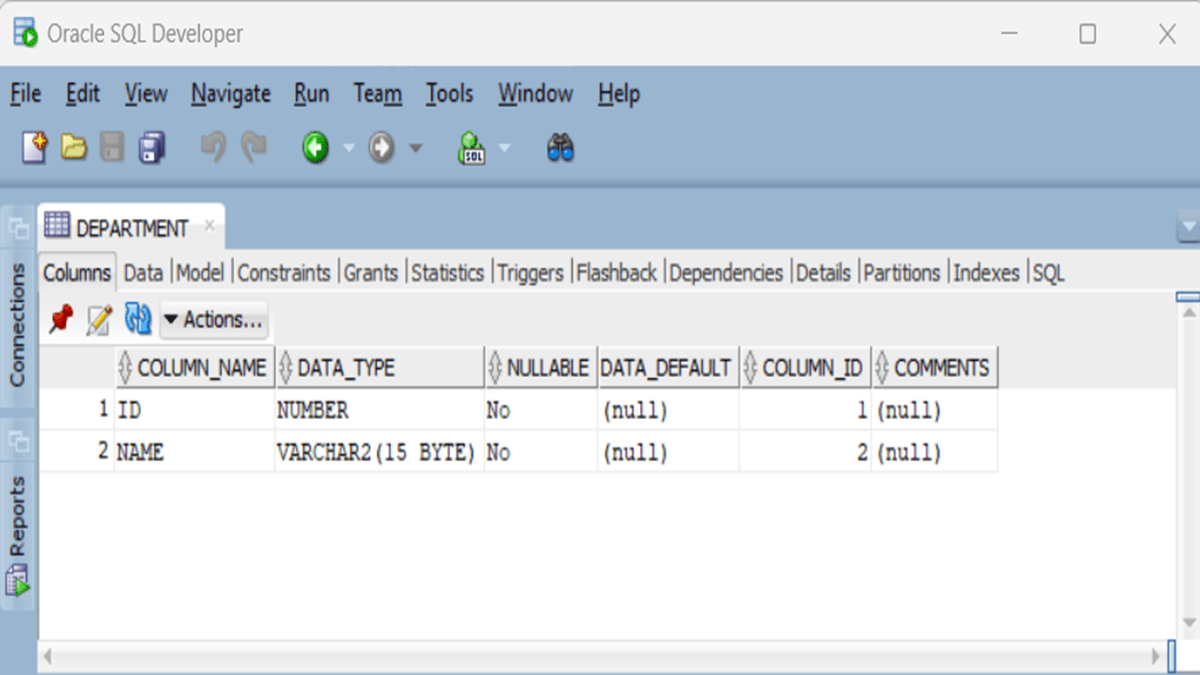# FahmidasClassroom

Learn by easy stepsThe concept of the second normal form (2NF) has been explained in this tutorial by using the Oracle database tables. Run the SQL Developer application and logged in with the valid username and password. You have to complete the tutorial of the 1NF before starting this tutorial.

Second Normal Form (2NF):

Run the following SQL statements to create a table named DEPARTMENT with two fields and insert two records into the table.

``````CREATE TABLE DEPARTMENT
(
ID NUMBER NOT NULL
, NAME VARCHAR2(15) NOT NULL
, CONSTRAINT DEPARTMENT_PK PRIMARY KEY (ID)
);

INSERT INTO "SYSTEM"."DEPARTMENT" (ID, NAME) VALUES ('1', 'CSE');
INSERT INTO "SYSTEM"."DEPARTMENT" (ID, NAME) VALUES ('2', 'BBA');

The table structure will be looked like the following image.``````

Run the following SQL statement that will create a new table named STD_DEPT2. The table will contain two primary keys and these are the primary keys of the STD_BASIC and DEPARTMENT tables. So, these keys are the foreign keys of this table.

``````
CREATE TABLE STD_DEPT2
(
STD_ID NUMBER NOT NULL
, DEPT_ID NUMBER NOT NULL
, CONSTRAINT STD_DEPT2_PK PRIMARY KEY (STD_ID, DEPT_ID)
, CONSTRAINT STD_DEPT2_FK1 FOREIGN KEY (STD_ID)
REFERENCES STD_BASIC (ID ) ON DELETE CASCADE
, CONSTRAINT STD_DEPT2_FK2 FOREIGN KEY (DEPT_ID)
REFERENCES DEPARTMENT( ID) ON DELETE CASCADE
);
``````

Run the following SQL statement that will insert 5 records into the STD_DEPT2 table that will work as the alternative of the STD_DEPT table that has been created in the previous tutorial.

``````INSERT INTO "SYSTEM"."STD_DEPT2" (STD_ID, DEPT_ID) VALUES ('1', '1');
INSERT INTO "SYSTEM"."STD_DEPT2" (STD_ID, DEPT_ID) VALUES ('2', '1');
INSERT INTO "SYSTEM"."STD_DEPT2" (STD_ID, DEPT_ID) VALUES ('3', '1');
INSERT INTO "SYSTEM"."STD_DEPT2" (STD_ID, DEPT_ID) VALUES ('4', '2');
INSERT INTO "SYSTEM"."STD_DEPT2" (STD_ID, DEPT_ID) VALUES ('5', '2');``````

The content of three tables will be looked like the following image.

The data redundancy has been removed by creating the third table and applying 2NF.

Third normal form (3NF)